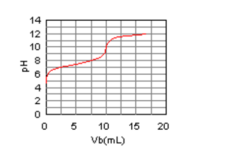# Problem: The curve for the titration of 50.0 mL of 0.0200 M HClO(aq) with 0.100 M NaOH(aq) is given below. Estimate the pKa of HClO.a) 7.0b) 10.0c) 12.0d) 5.0e) 7.5

###### FREE Expert Solution
95% (365 ratings)
###### Problem Details

The curve for the titration of 50.0 mL of 0.0200 M HClO(aq) with 0.100 M NaOH(aq) is given below. Estimate the pKa of HClO.

a) 7.0

b) 10.0

c) 12.0

d) 5.0

e) 7.5What scientific concept do you need to know in order to solve this problem?

Our tutors have indicated that to solve this problem you will need to apply the Acid and Base Titration Curves concept. You can view video lessons to learn Acid and Base Titration Curves. Or if you need more Acid and Base Titration Curves practice, you can also practice Acid and Base Titration Curves practice problems.

What is the difficulty of this problem?

Our tutors rated the difficulty ofThe curve for the titration of 50.0 mL of 0.0200 M HClO(aq) ...as low difficulty.

How long does this problem take to solve?

Our expert Chemistry tutor, Jules took 1 minute and 48 seconds to solve this problem. You can follow their steps in the video explanation above.

What professor is this problem relevant for?

Based on our data, we think this problem is relevant for Professor Rowntree's class at University of Guelph.